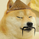6666... 6+6+6+6 = 24 => 6
618... 6+1+8 = 7+8 = 1+5 => 6
666 ==> 1+8 ==> 9 The most yang, most powerful number... also the age of bitcoin